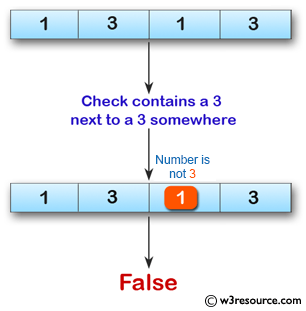﻿ Swift Array Programming Exercise: Check if a given array of integers contains a 3 next to a 3 somewhere - w3resource# Swift Array Programming Exercises: Check if a given array of integers contains a 3 next to a 3 somewhere

## Swift Array Programming: Exercise-27 with Solution

Write a Swift program to check if a given array of integers contains a 3 next to a 3 somewhere.

Pictorial Presentation:Sample Solution:

Swift Code:

``````func has33(array_nums: [Int]) -> Bool {
for x in 0..<array_nums.count-1
{
if array_nums[x] == 3 && array_nums[x+1] == 3
{
return true
}
}
return false
}
print(has33(array_nums: [1, 3, 3]))
print(has33(array_nums: [1, 3, 1, 3]))
print(has33(array_nums: [3, 1, 3, 3]))
```
```

Sample Output:

```true
false
true
```

Swift Programming Code Editor:

Improve this sample solution and post your code through Disqus

What is the difficulty level of this exercise?

﻿